# How to Calculate Days, Weeks, Months and Years between Two Dates

This post will teach you how to calculate days, weeks, months and years between two dates in excel. How do I count the number of days, weeks, months and years between 2 dates in excel.

## Calculate days between two dates

If you want to calculate the difference in days between two dates, you can use the DATEDIF function to create an excel formula as follows:

`=DATEIF(B1,B2, "D")`

This formula will calculate days between two dates in cell B1 and B2, then returns the value in days.

## Calculate months between two dates

If you want to calculate the difference in months between tow dates, you can also use the DATEDIF function to create the following generic formula:

`=DATEDIF(B1,B2,"M")`

You should note that the third argument is “M” in the DATEDIF function. So this formula returns the value in months between two dates in excel.

## Calculate years between two dates

You can also use the DATEDIF function to calculate the number of years between two dates in excel, just refer to the following excel formula based on the DATEDIF function:

`=DATEDIF(B1,B2,"Y")`

This formula returns the value in years.

## Calculate weeks between two dates

If you want to calculate the number of weeks between two dates, you just need to subtract start date from the end date and then the returned result is divided by 7. So you can write the below generic formula:

`=(B2-B1)/7`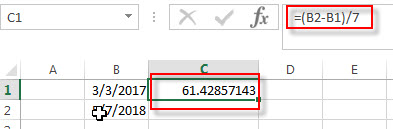This formula will return a decimal number, you can change the number format as you need.

Or you can use the INT function to rounds down to the nearest whole number or use ROUND function to round to nearest whole number.

=INT((B2-B1)/7)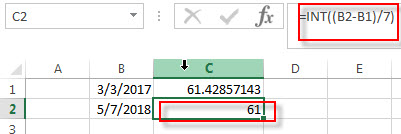=ROUND((B2-B1)/7,0)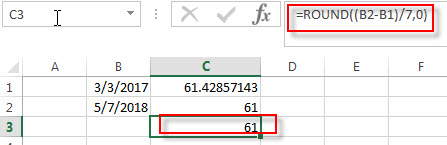You may be want to display the word “week” before the week number in the cell, you can do it as following steps:

1# right-click on the selected cells, then select Format Cells

2# on the Number Tab, choose Custom under Category, then select # “weeks” type.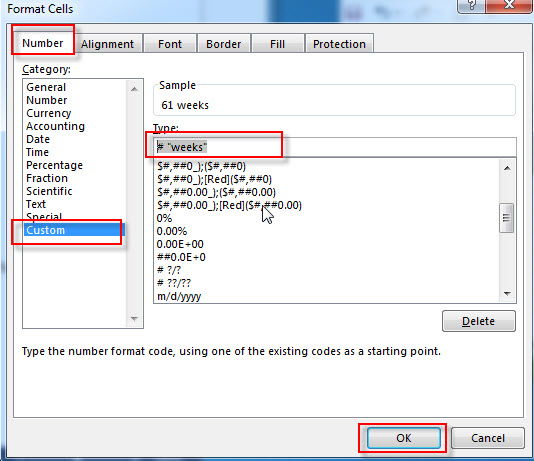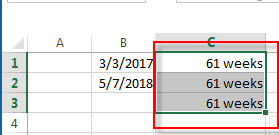## Calculate Years, Months and Days between two dates

If you want to determine how many years, months and days between two dates, you can use the DATEDIF function to create the following complex formula:

`=DATEDIF(B1,B2,"Y") & " Years, " & DATEDIF(B1,B2,"YM") & " Months, " & DATEDIF(B1,B2,"MD") & " Days"`

If you do not want to use the DATEDIF function, and you can also use the following formula to achieve the same result:

`=INT((TODAY()-A1)/365.25) & ” years , ” & INT(MOD((TODAY()-A1)/365.25,1)*12) & ” months and ” & INT(MOD((TODAY()-A1)/30.4375,1)*30.4375) & ” days”`

### Related Functions

• Excel INT function
The Excel INT function returns the integer portion of a given number. And it will rounds a given number down to the nearest integer.The syntax of the INT function is as below:= INT (number)…
• Excel DATEDIF function
The Excel DATEDIF function returns the number of days, months, or years between tow dates.The syntax of the DATEDIF function is as below:=DATEDIF (start_date,end_date,unit)…
• Excel TODAY function
The Excel TODAY function returns the serial number of the current date. So you can get the current system date from the TODAY function. The syntax of the TODAY function is as below:=TODAY()…
• Excel Round function
The Excel INT function rounds a number to a specified number of digits. You can use the ROUND function to round to the left or right of the decimal point in Excel.The syntax of the ROUND function is as below:=ROUND (number, num_digits)…